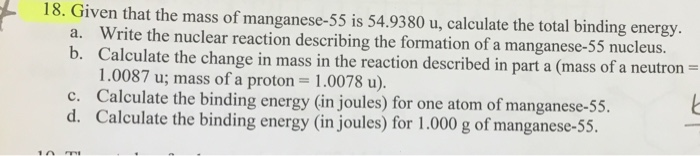# Solved: 18. Given That The Mass Of Manganese-55 Is 54.9380 U, Calculate The Total Binding Energy A. Write The Nuclear Reaction Describing The Formation Of A Manganese-55 Nucleus. B. Calculate The Chan

By |18. Given that the mass of manganese-55 is 54.9380 u, calculate the total binding energy a. Write the nuclear reaction describing the formation of a manganese-55 nucleus. b. Calculate the change in mass in the reaction described in part a (mass of a neutron 1.0087 u; mass of a proton 1.0078 u). c. Calculate the binding energy (in joules) for one atom of manganese-55 d. Calculate the binding energy (in joules) for 1.000 g of manganese-55 10